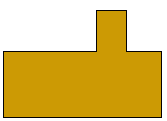Name: ___________________Date:___________________

 Email us to get an instant 20% discount on highly effective K-12 Math & English kwizNET Programs!

### MEAP Preparation - Grade 7 Mathematics1.145 Geometry Part Three - Review Test

 Q 1: The formula for perimeter of a rectangle with length L and width W is given byP = 2 x (L+W)P = 2 x (LxW)P = 2 + (L+W) Q 2: In the figure name the location or ordered pair of point C.(5,4)(1,3)(5,3) Q 3: Is this figure symmetric?NoYes Q 4: A soda can looks most like a ______.cylinderconesquare Q 5: What is the area of a rectangle with length 5 inches and width 9 inches45 sq inches4 sq inches14 sq inches Q 6: A sphere looks most like which object?eraserclockballglass Q 7: What is the volume of a cube with 2 inches.8 cubic inches12 cubic inches16 cubic inches Q 8: Is this figure symmetric?NoYes Question 9: This question is available to subscribers only! Question 10: This question is available to subscribers only!